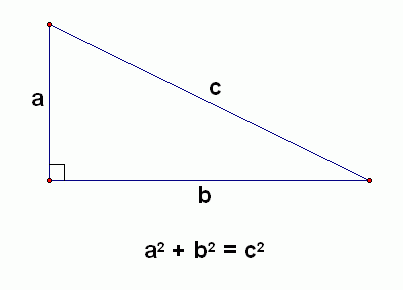# Practice on Toph

Participate in exhilarating programming contests, solve unique algorithm and data structure challenges and be a part of an awesome community.

# Pythagoras Rocks

Limits 1s, 512 MB

A Pythagorean triple consists of three positive integers $a$, $b$, and $c$, such that $a^2 + b^2 = c^2$.

The Pythagoras's theorem says, for a right triangle (a triangle with one of the sides being $90\degree$), the same formula holds (where $a$, $b$, and $c$) are the three sides of the triangle.In this problem, you will be given a Pythagorean triple, you will have to determine which of the three numbers in the triple represents the hypotenuse of the triangle.

## Input

The only input line contains three integers $X$, $Y$, and $Z$ ($1 \le X, Y, Z \le 10^9$), the three sides of a Pythagorean triangle.

## Output

Print the number among $X$, $Y$, and $Z$ that represents the hypotenuse.

## Samples

InputOutput
3 4 5

5

InputOutput
5 12 13

13SortinguDebug

### Statistics

93% Solution Ratio

subintra2016.8$8KpTbEarliest, Nov '16 subintra2016.13$V5M33Fastest, 0.0s

nishat19Lightest, 0 B

touhidurrrShortest, 36B

### Submit

 Replay of SUB Intra University Programming Contest 2016Dec '16 SUB Intra University Programming Contest 2016Nov '16 Intra KUET Programming Contest 2016Dec '16 BRACU Intra Programming Contest Fall 2018 (Junior)Sep '18 JnU Intra-Department Programming Contest 2018 (Junior Division)Oct '18 Intra KUET Programming Contest 2019 MockApr '19